Giỏ hàng

## How to Design the Transformer in a Cuk Converter

Basics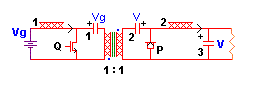The voltage impressed across the primary side winding during D is just the voltage on the input side energy transfer cap. [Q is ON, P is OFF]

But since the input inductor and primary transformer winding cannot stand off DC, the (steady-state, average, or DC) voltage on this capacitor is just Vg, the source voltage. This means that the primary winding sees DVgTs volt-seconds each time Q is ON. Likewise, the secondary sees D’VTs when P is ON (and Q is OFF.)

Note That: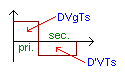…if the magnetizing inductance is not to saturate, i.e. the transformer’s core is not to ‘walk off’ the top of the B-H loop, DVgTs must equal D’VTs. But this is just a restatement of the relation V/Vg=D/D’, which is automatic!
Net Excitation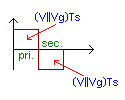Now, one of the reasons for the variable duty cycle in a switcher is to correct for various input voltages. That is, our duty cycle changes depending on Vg, to maintain a constant output voltage.

But if D is a function of Vg, and the volt-seconds across the primary winding are just DVgTs, we should be able to find a simple relation by eliminating D!

Considering first a 1-to-1 transformer, we remember that V/Vg = D/D’ = M, where V is the output voltage. Using the relations D=M/(1+M) and D’=1/(1+M); and rewriting DVg {=D’V} as MVg/(1+M) {=V/(1+M)} we reach the surprising but altogether satisfactory conclusion that DVg = (V||Vg)!

Adjustment When the Turns Ratio is Not One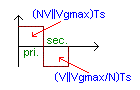If the design requires a transformer to step down, with N turns at the primary side to each turn of the secondary, the expression becomes DVgTs = (NV||Vg). Naturally, any output can be used, so long as the N corresponds to the particular V chosen. Note that when stepping up, N will be <1! In general, if there are Np turns at the primary side for each Ns turns at the secondary: N = Np/Ns.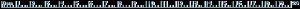Efficiency

To account for inefficiencies, replace N with N/% where % is the estimated efficiency of the finished converter, not including housekeeping, control, or drive circuits.

In a multi-output converter, also exclude losses in the outputs not being used to calculate volt-second excitation. Remember that only one V and N need be used in this calculation. The one with the greatest output power is usually the best to use!

Practically Speaking

As explained on the old site, transient current must be provided for in designing the transformer of the Cuk Converter. [For a discussion of this circumstance, click on the icon above.]

The solution turns out to be simple, though a considerable calculation is required to reach it! Introducing a gap in the core to reduce its permeability to mu = 300 or so does the trick. Remember that the (effective) mu=lm/lg, where lm is the magnetic path length of the transformer, while lg is the gap length. Thus a transformer with a 3cm path length takes a 4 mil gap, or 2 mils in each leg!

2,430,000₫

1,050,000₫

1,900,000₫

Liên hệ

550,000₫